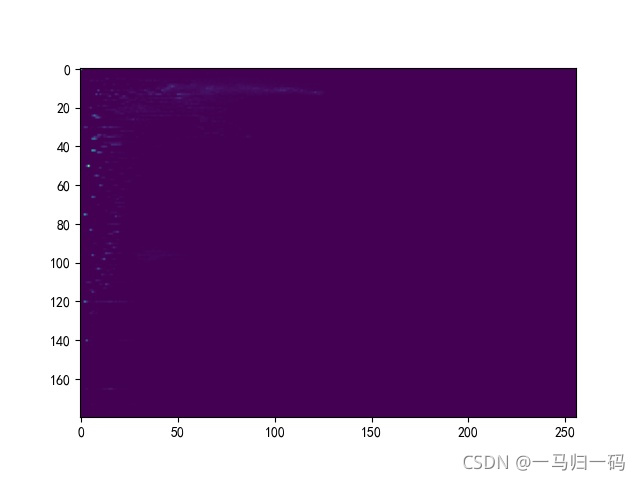﻿ Python+OpenCV图像处理之直方图统计_python_脚本之家
python# Python+OpenCV图像处理之直方图统计

BINS

DIMS

RANGE

## 2. 直方图绘制

### （1）读取图像信息

```"""
Author:XiaoMa
date:2021/10/24
"""
#调用需要的包
import cv2
import matplotlib.pyplot as plt

img0 = cv2.imread('E:\From Zhihu\For the desk\cvseven.jpeg')
img1 = cv2.cvtColor(img0, cv2.COLOR_BGR2GRAY)     #转化为灰度图
h, w = img1.shape[:2]
print(h, w)
cv2.namedWindow("W0")
cv2.imshow("W0", img1)
cv2.waitKey(delay = 0)```

419 636### （2）绘制直方图

hist = cv2.calcHist(images, channels, mask, histSize, ranges, accumulate)

images:原图

channels:指定通道 代表灰度图，如果读入的图像不是灰度图，该值可以是,,分别代表通道 B,G,R

histSize:BIN 的数量

ranges:像素值范围

accumulate:累计标识，一般可以省略

```#绘制直方图
hist = cv2.calcHist([img1], , None, , [0, 255])
plt.plot(hist, color = 'lime', label = '直方图', linestyle = '--')
plt.legend()
plt.savefig('E:\From Zhihu\For the desk\cvseven1.jpeg')
plt.show()``````"""
Author:XiaoMa
date:2021/10/24
"""
#调用需要的包
import cv2
import matplotlib.pyplot as plt

plt.rcParams['font.family'] = 'SimHei'       #将全局中文字体改为黑体

img0 = cv2.imread('E:\From Zhihu\For the desk\cvseven.jpeg')
img1 = cv2.cvtColor(img0, cv2.COLOR_BGR2GRAY)
h, w = img1.shape[:2]
print(h, w)
cv2.namedWindow("W0")
cv2.imshow("W0", img0)
cv2.waitKey(delay = 0)

#绘制直方图
hist = cv2.calcHist([img0], , None, , [0, 255])
plt.plot(hist, color = 'lime', label = '蓝色通道直方图', linestyle = '--', alpha = 1)
plt.legend()
plt.savefig('E:\From Zhihu\For the desk\cvseven1.jpeg')
plt.show()```## 3. 掩膜直方图

### （2）实现代码

```##得到掩膜图
cv2.namedWindow("W1")
cv2.imshow("W1", img0_1)
cv2.waitKey(delay = 0)``````#绘制掩膜直方图
##得到掩膜图
cv2.namedWindow("W1")
cv2.imshow("W1", img1_1)
cv2.waitKey(delay = 0)
##绘制掩膜直方图和部分图像直方图
hist1 = cv2.calcHist([img1], , mask, , [0, 255])         #掩膜图直方图，参数需要修改
hist2 = cv2.calcHist([img1], , None, , [0,255])
plt.plot(hist1, color = 'b', label = '掩膜直方图', linestyle = '--')
plt.plot(hist2, color = 'r', label = '原图直方图', linestyle = '-.')
plt.legend()
plt.savefig('E:\From Zhihu\For the desk\cvseven2.jpeg')
plt.show()```## 4. H-S 直方图

### （1）基本概念

H(Hue) - S(Saturation) 直方图，即色调 - 饱和度直方图

```img0_2 = cv2.cvtColor(img0, cv2.COLOR_BGR2HSV) #将 RGB 空间转化为 HSV 空间
cv2.namedWindow("W2")
cv2.imshow("W2", img0_2)
cv2.waitKey(delay = 0)```### （2）绘制二维H-S直方图

```##绘制H-S直方图
hist3 = cv2.calcHist ([img0_2], [0, 1], None , [180, 256], [0, 180, 0, 256])#官网给出的解释：channel = [0,1] 因为我们需要同时处理 H 和 S 平面；bins = [180,256] H 平面为 180，S 平面为 256；range = [0,180,0,256] 色调值介于 0 和 180 之间，饱和度介于 0 和 256 之间
plt.imshow(hist3)
plt.savefig('E:\From Zhihu\For the desk\cvseven3.jpeg')
plt.show()```